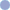LaplacianThe Laplacian filter is used for detection of edges in an image. It highlights areas in which intensity changes rapidly producing a picture of all the edges in an image.
 SettingsName Default DescriptionRadius 0.8 pixels This value determines the size of the edges that are detected. This value represents the standard deviation of the Laplacian of Gaussian function.How it WorksThe Laplacian filter is a standard Laplacian of Gaussian convolution. This is a second derivative function designed to measure changes in intensity without being overly sensitive to noise. The function produces a peak at the start of the change in intensity and then at the end of the change. Because the Laplacian of Gaussian produces a fairly wide convolution for a small radius this filter can become quite computationally expensive as radius is increased.
 ExampleThe following examples show the effect of a Laplacian filter applied with a number of different settings.Original Image before Laplacian FilterLaplacian Radius 0.8 PixelsLaplacian Radius 1.6 PixelsLaplacian Radius 3.2 Pixels